Next: 6 Circles
Up: 5 Polygons

# 5.3 Regular Polygons

A polygon is regular if all its sides are equal and all its angles are equal. Either condition implies the other in the case of a triangle, but not in general. (A rhombus has equal sides but not necessarily equal angles, and a rectangle has equal angles but not necessarily equal sides.)

For a k-sided regular polygon of side a, let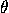be the angle at any vertex, r and R the radii of the inscribed and circumscribed circles (r is called the apothem). As usual, let ska be the half-perimeter. Then: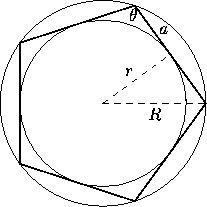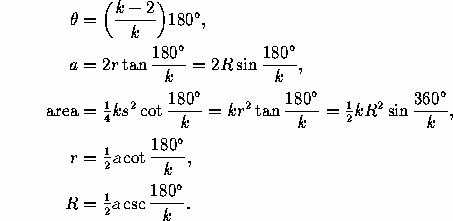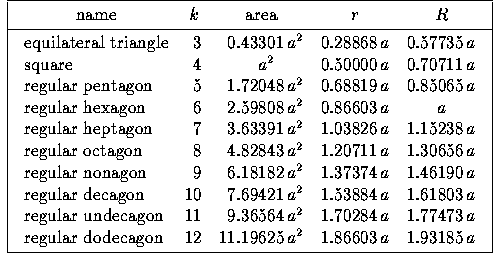If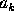denotes the side of a k-sided regular polygon inscribed in a circle of radius R, we have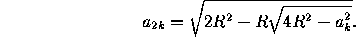Ifdenotes the side of a k-sided regular polygon circumscribed about the same circle, we have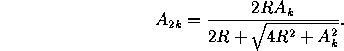In particular,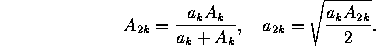The areas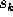,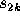,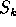andof the same polygons satisfy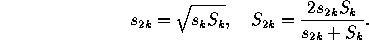Next: 6 Circles
Up: 5 PolygonsThe Geometry Center Home Page